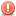# Thread: How to use calculated column value in where clause

1.Registered User
Join Date
Sep 2010
Posts
2

##How to use calculated column value in where clause

I need to retrieve data filtered by the results of a calculated column - how?

INFORMIX:

SELECT

TUD_PK_LIST_NO AS PKL,
TUP_TAG_NO AS TAG,
(
CASE
(
CASE (SELECT ttt_prnt_tag_no FROM INTTTT_REC WHERE ttt_itm_ctl_no = tup_itm_ctl_no) WHEN NULL THEN (SELECT MIN(pkc_cmpt_tag_no) FROM INTPKC_REC WHERE pkc_pkg_ctl_no = tup_itm_ctl_no GROUP BY pkc_pkg_ctl_no) ELSE (SELECT ttt_prnt_tag_no FROM INTTTT_REC WHERE ttt_itm_ctl_no = tup_itm_ctl_no) END )
WHEN NULL
THEN (SELECT TRIM(tag_mst_tag_no) FROM INTTAG_REC WHERE tag_itm_ctl_no = tup_itm_ctl_no)
ELSE
(
CASE
(
SELECT tag_mst_tag_no
FROM
INTTAG_REC
WHERE tag_itm_ctl_no =
(
CASE (SELECT ttt_itm_ctl_no FROM INTTTT_REC WHERE ttt_itm_ctl_no = tup_itm_ctl_no)
WHEN NULL
THEN (SELECT MIN(pkc_cmpt_ctl_no) FROM INTPKC_REC WHERE pkc_pkg_ctl_no = tup_itm_ctl_no GROUP BY pkc_pkg_ctl_no)
ELSE (SELECT ttt_itm_ctl_no FROM INTTTT_REC WHERE ttt_itm_ctl_no = tup_itm_ctl_no)
END
)

)
WHEN NULL
THEN (SELECT TRIM(atg_mst_tag_no) FROM INAATG_REC WHERE atg_itm_ctl_no =
(
CASE (SELECT ttt_itm_ctl_no FROM INTTTT_REC WHERE ttt_itm_ctl_no = tup_itm_ctl_no)
WHEN NULL
THEN (SELECT MIN(pkc_cmpt_ctl_no) FROM INTPKC_REC WHERE pkc_pkg_ctl_no = tup_itm_ctl_no GROUP BY pkc_pkg_ctl_no)
ELSE (SELECT ttt_itm_ctl_no FROM INTTTT_REC WHERE ttt_itm_ctl_no = tup_itm_ctl_no)
END
)
)
ELSE
(
SELECT TRIM(tag_mst_tag_no)
FROM
INTTAG_REC
WHERE tag_itm_ctl_no =
(
CASE (SELECT ttt_itm_ctl_no FROM INTTTT_REC WHERE ttt_itm_ctl_no = tup_itm_ctl_no)
WHEN NULL
THEN (SELECT MIN(pkc_cmpt_ctl_no) FROM INTPKC_REC WHERE pkc_pkg_ctl_no = tup_itm_ctl_no GROUP BY pkc_pkg_ctl_no)
ELSE (SELECT ttt_itm_ctl_no FROM INTTTT_REC WHERE ttt_itm_ctl_no = tup_itm_ctl_no)
END
)
)
END
)
END
) AS MASTER,

FROM BLA BLA BLA
WHERE `MASTER` = '123456'

hello@worddaily.comReply With Quote

2.Registered User
Join Date
Aug 2022
Posts
22
There are a few ways to retrieve data filtered by the results of a calculated column it totally depends on the database you are using. Here are some examples:
1. SQL: You can use a SELECT statement with a WHERE clause to filter the results based on a calculated column. For example, if you have a table named "orders" with a calculated column named "total_cost," you can retrieve all orders where the total cost is greater than 100 with the following query:

SELECT * FROM orders WHERE total_cost > 100;

2. Python and Pandas: If you are using Python and the Pandas library to manage your data, you can use the query() method to filter the results based on a calculated column. For example, if you have a DataFrame named "df" with a calculated column named "total_cost," you can retrieve all rows where the total cost is greater than 100 with the following code:

df.query('total_cost > 100')

Depending on the database management system and programming language the commands may vary.Reply With Quote

####Posting Permissions

• You may not post new threads
• You may not post replies
• You may not post attachments
• You may not edit your posts
•